# Assignments

In general, inequalities with fractions are difficult to solve. In order to solve such inequalities we use so-called 'number lines'.
It is very important to notice that it is very tricky to use cross-multiplication and it is highly discouraged to apply this popular technique with inequalities, see also Equations with fractions.

1. Solve: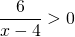Solution

2. Solve:Solution

3. Solve: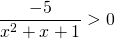Solution

4. Solve: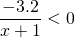Solution

5. Solve: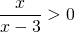Solution

6. Solve: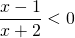Solution

7. Solve: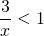Solution

8. Solve: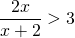Solution

9. Solve: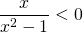Solution

10. Solve: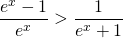Solution

0